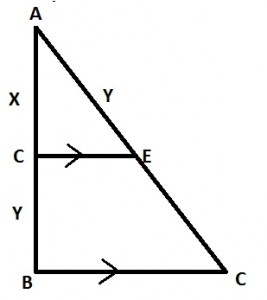In the diagram, DE||BC, |AD| = x cm and |DB| = |AE| = ycm. Find |CE| in terms of x and y

A. x
B. x^2/y
C. y/x^2
D. y^2/x

Correct Answer: Option D

D. y^2/x

Explanation
x/x+y=y/x+CE

xy+xCE=xy+y^2

∴xCE=y^2

CE=y^2/x